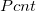# Center Pivot Movement Costs

Calculates the electric energy costs to move a center pivot around in a circle for a season. Does not include pumping energy costs. Requires an estimate of the irrigation system operating hours per season. The lessons from this calculator are that the movement costs are small compared to the potential return from the crop, but that a lower % setting saves money (same water applied, less movement). Slower pivots are more efficient with the water as well (less evaporation losses from a wet canopy). General guidelines are to slow the pivot down until you start having problems with runoff, then adjust it up a little. Learn more about the units used on this page.

Number of Pivot Towers:
Pivot Tower Drive Motor Size:
Pivot Average % Setting:
%
hours
Electric Energy Cost (\$/kWh):
\$/kWh
Pivot Movement Costs per Season (\$):
\$

## The EquationsWhere:= Irrigation system operating hours per season (hrs)= Average pivot % setting (decimal, or %/100)= Number of pivot towers. Assumes equal spacing between towers (not always true, but it will get you close).= Pivot tower drive motor size (hp).= Electric motor efficiency (decimal). This calculator assumes a 78% efficiency.

WSU Prosser - IAREC, 24106 N Bunn Rd, Prosser WA 99350-8694, 509-786-2226. Contact Us